1 Symmetric Ties and Undirected Graphs

nodes and edges are indeed the building blocks of a graph. However, types of relationships that the edges represent can change both how we understand the network conceptually and also what mathematical techniques we can apply to the graph when we compute graph metrics (the subject of a future lesson). The basic idea is that when we do network analysis, we want to map our understanding of the nature of the social relationships we are studying to the types of graphs we use to represent the network formed by the concatenation of those relationships.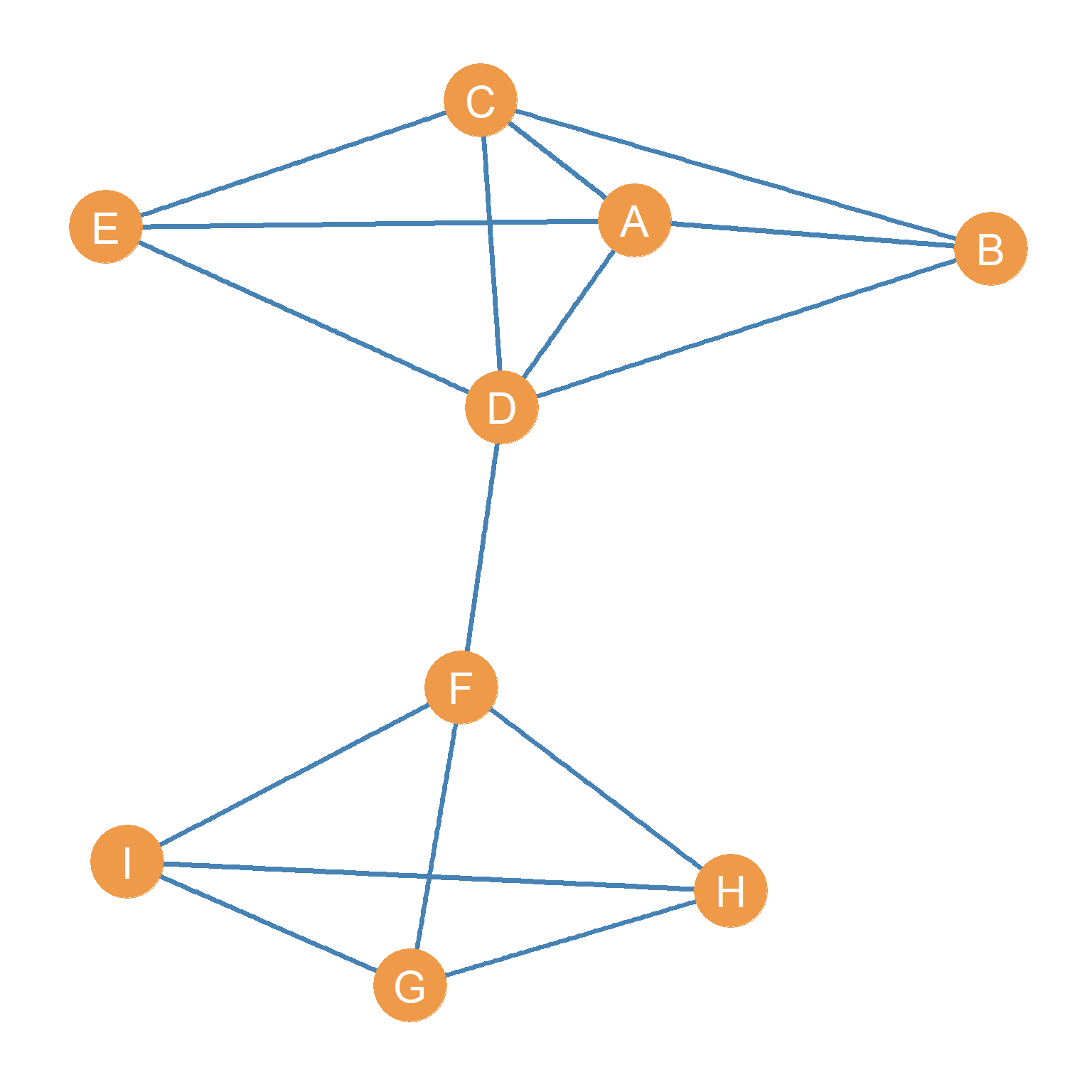Figure 1.1: A undirected graph

Let us assume that Figure 1.1 represents a network of people who spend time together. One way of building this network would be to ask people on your dorm room floor who are the people that they spend some amount of time (e.g., more than an hour a week) hanging out with. By definition the relation “spending time together” lacks any inherent directionality. Mutuality (or reciprocity) is built in by construction. It would be nonsensical for a person (say A) to claim that they spend time with another person (say B) and for B to say that they do not spend time with A. In social network analysis these types of ties are called symmetric ties.

Accordingly, two people being in the same place at the same time (co-location), even if they do not one another, is an example of a symmetric tie. You also have the symmetric tie “being in the same class as” every other student that is also taking your Social Networks seminar this term. Note that, in this sense, all co-memberships (e.g., being in the same club or organization or being part of the same family) create symmetric ties among all actors involved (we will revisit this topic when talking about two-mode networks in another lesson). If I am a member of your family, you are also my family member; if we are both members of the soccer club, we are considered teammates. Social networks composed of symmetric ties are represented using undirected graphs like the one shown in Figure 1.1.

Networks composed of symmetric ties have some interesting properties. If we know that the relationship (R) linking two nodes A and B is symmetric, then only a single edge exists that links them, and it does not matter whether we call this edge AB or BA. The order does not matter. In this way, we can formally define as symmetric tie as one that lacks directionality; if a tie is symmetric, then if we know that A is related to B (the AB edge is part of the edge set of the graph), then we know by necessity that B is related to A.

Can you think of other examples of symmetric ties? Is friendship, as culturally defined in the contemporary world, a symmetric tie?

2 Asymmetric Ties and Directed Graphs

In contrast to spending time together, being members of the same family, or being in the same place at the same time, some social ties allow for inherent directionality. Edges in these graphs are are called asymmetric ties. That is, one member of the pair can claim to have a particular type of social relationship with the other, but it is possible (although not necessary) that the other person fails to have the same relationship with the first.

Helping or social support relations, are like this. For instance, you can help someone with their homework, or given them personal advice, but this does not necessarily mean that that person will return the favor. They may, or they may not. The point is that, in contrast to symmetric tie, mutuality or reciprocity is not built in by definition, but must happen as an empirical event in the world. We need to ask the other person to find out (or check their email logs). Can you think of other examples of asymmetric social ties?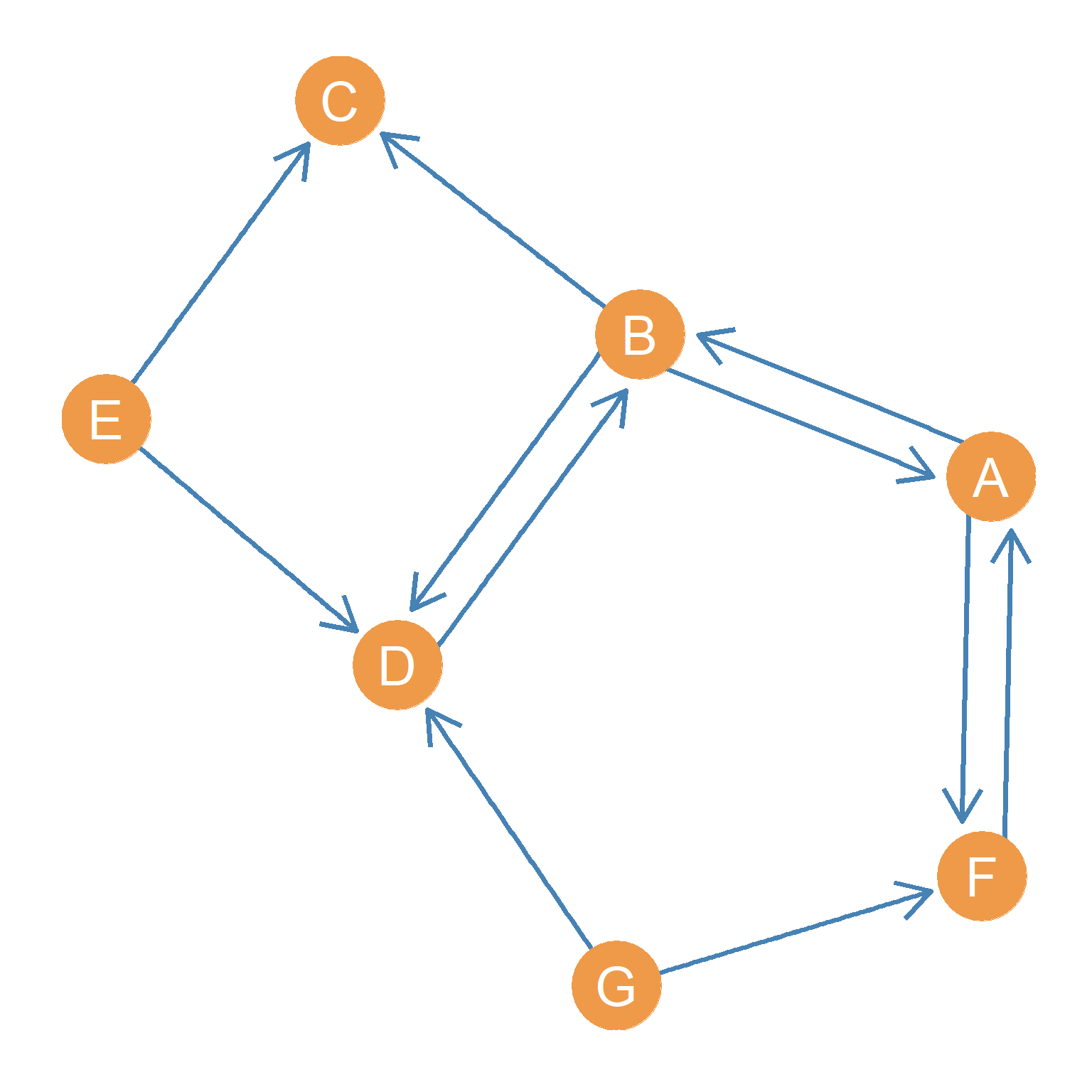Figure 2.1: A directed graph.

Reciprocity is an important concept in social network analysis. Some have said it is perhaps the most important concept for understanding human society (Gouldner 1960Gouldner, Alvin W. 1960. “The Norm of Reciprocity: A Preliminary Statement.” American Sociological Review, 161–78.), which may be a bit of an exaggeration. Only asymmetric ties may have the property of being non-reciprocal or having more or less reciprocity. If I think you are my friend, I very much hope that you also think you are my friend. That said, sociologists have found that in many natural social settings this is not the case. Sometimes people think they are friends with others, but those other people disagree (Carley and Krackhardt 1996Carley, Kathleen M, and David Krackhardt. 1996. “Cognitive Inconsistencies and Non-Symmetric Friendship.” Social Networks 18 (1): 1–27.). For this reason, sociologists typically ask: if I do you a favor, would you do me a favor in the future? Additionally, sociologists often ask: if I treat you with respect, will you also treat me with respect? If I text you, will you text me back? If this is true, we have a level of reciprocity in our relationship.

For some ties, such advice or support, or friendship relations, reciprocity is all or none; it either exists or it does not. For instance, the friendship offer you extend to someone may be reciprocated (or not). In the same way, you can like someone and they may like you back (or not), like the notes you passed around in middle school. For other ties, such as communication ties (e.g., those defined by the amount of texting, or calling), reciprocity is a matter of degree, there may be more or less. For instance, you can text someone 10 times a day, but they may text you back only half of those instances. In all cases, reciprocity is at a maximum when the content of the relationship is equally exchanged between actors.

Can you think of relationships in your life characterized by more or less reciprocity?

Just like symmetric ties are represented using a particular type of graph (namely, an undirected graph), social networks composed of asymmetric ties are best represented by a type of graph called a directed graph.1 Directed graphs are also called digraphs Figure 2.1 shows the point and line diagram picture of a directed graph. What were simple lines for an undirected graph (Figure 1.1) have been replaced with arrows indicating directionality. A node sends a relationship to the node that the arrow points to. Up to two directed arrows may link nodes going both ways.

If Figure 2.1 were an advice network (Cross, Borgatti, and Parker 2001Cross, Rob, Stephen P Borgatti, and Andrew Parker. 2001. “Beyond Answers: Dimensions of the Advice Network.” Social Networks 23 (3): 215–35.), on the other hand, we could say that H seeks advice from D, but D does not seek advice from H. This may be because D is higher in the office hierarchy or is more experienced than H, in which case lack of reciprocity may be indicative of an authority relationship between the two nodes.

In a directed graph, for every edge, there is a source node and a destination node. So in the case of “A helps B” the source node is A and the destination node is B. In the case of “B helps A” the source node is B and the destination node is A. This means that in a directed graph, in contrast to a undirected one, the order in which you list the nodes when you name the edges matters. Thus, the edge AB is a different one from the edge BA. For instance, the first one may exist but the second one may not exist.

One must always be careful when examining a directed network to make sure one properly understands the direction of the underlying social relationships!

2.1 Node Neighborhoods in Directed Graphs

Just like in undirected (simple) graphs, each node in a directed graph has a node neighborhood. However, because now each node can be the source or destination for a asymmetric edges, this means that we have to differentiate the neighborhood of a node depending on whether the node is the sender or the recipient of a given link.

So, we say that a node j is an an in-neighbor of a node i if there is a directed link with j as the source and i as the destination node. For instance, in Figure 2.1, E is an in-neighbor of C, because there’s a asymmetric edge with E as the source and C as the destination.

In the same way, we say that a node i is an out-neighbor of a node j if there is a directed link with i as the source and j as the destination. For instance, in Figure 2.1, F is an in-neighbor of G, because there’s a asymmetric edge with G as the source and F as the destination

For each node, the full set of in-neighbors forms the in-neighborhood of that node. This is written $$N^{in}(v)$$, where $$v$$ is the label corresponding to the node. For instance, in Figure 2.1, the node set $$N^{in}(D) = \{B, E, G\}$$ is the in-neighborhood of node D.

In the same way, the full set of in-neighbors defines the out-neighborhood of that node. This is written $$N^{out}(v)$$, where $$v$$ is the label corresponding to the node. For instance, in Figure 2.1, the node set $$N^{out}(B) = \{A, C, D\}$$ is the out-neighborhood of node B.

Note that typically, the set of in-neighbors and out-neighbors of a given node will not be exactly the same, and sometimes the two sets will be completely disjoint (they won’t share any members).

Nodes will only show up in both the in and out-neighborhood set when there are reciprocal or mutual ties between the nodes. For instance, in Figure 2.1, the out-neighborhood of node F is $$\{A\}$$ and the in-neighborhood is $$\{A, G\}$$. Here node A shows up in both the in and out-neighborhood sets because A has a reciprocal tie with F.

2.2 Node Degree in Directed Graphs

Because in a directed graph, each node has two distinct set of neighbors, we can compute two versions of degree for the same node.

• in a directed graph, for any node i, we can count the number of edges that have a given node $$v$$ as their destination node. This is also the cardinality of the in-neighborhood set of that node. This is called a node’s indegree and it is written $$k^{in}_i$$, where i is the label corresponding to that node.

• Additionally, in a directed graph, for any node i, we can count the number of edges that have a given node $$i$$ as their source node. This is also the cardinality of the out-neighborhood set of that node. This is called that node’s outdegree and it is written as $$k^{out}_i$$, , where i is the label corresponding to that node.

For instance, in Figure 2.1, $$k^{out}_B = 3$$ and $$k^{in}_B = 2$$. Node B has three outgoing ties (from nodes A, C, and D) and three incoming ties (from nodes A and D).

Can you calculate what the indegree and outdegree of node D in Figure 2.1 is?

The graph theoretic ideas of indegree and outdegree have clear sociological interpretations. In a social network, for instance, a node having a large outdegree could indicate a sociable person (a person that likes to connect with others), while having a large indegree can indicate a popular person (e.g., a person lots of other people want to be friends with).2 In a later lesson we will see how to use a directed graph’s asymmetric adjacency matrix to readily compute the outdegree and indegree in real social networks.

This means that in a directed graph, there will typically be three types of (non-isolate) nodes (Harary, Norman, and Cartwright 1965Harary, Frank, Robert Z Norman, and Dorwin Cartwright. 1965. Structural Models: An Introduction to the Theory of Directed Graphs. Wiley.):

• First, there will be nodes that receive ties but don’t send them. These are called receivers (like node C in Figure 2.1). For receiver nodes $$k_{in} > 0$$ and $$k_{in} = 0$$.
• Second, there will be nodes that receive ties and also send out ties. These are called carriers (like nodes A and B in Figure 2.1). For carrier nodes, $$k_{in} > 0$$ and $$k_{in} > 0$$.
• Finally, there will be nodes that send ties but don’t receive them. These are called transmitters (like nodes E and G in Figure 2.1). For transmitter nodes, $$k_{in} = 0$$ and $$k_{out} > 0$$.

3 Anti-Symmetric Ties and Tree Graphs

There is a particular type of directed relationship that has the property of only going in one direction. These are called anti-symmetric ties. Like asymmetric ties, anti-symmetric ties have a directionality (and thus source and destination nodes), but reciprocity is forbidden by definition. That means that if A is anti-symmetrically connected to B, then B cannot send the same type of tie back to A (although B may be connected, and typically is, to A via some other type of tie in a different network).

A common example of anti-symmetric ties in political sociology are patron-client ties (Martin 2009Martin, John Levi. 2009. Social Structures. Princeton University Press.). Patrons can have many clients, but it is impossible for client of a patron to also be a patron to the same person. Other types of anti-symmetric ties are hierarchical relations at work, and cross-generation links in families. Your boss is your boss, while you are not your boss’ boss. In armies and other command and control structures, giving orders to is an anti-symmetric relation. An officer who gives orders to another officer (and thus commands them) cannot by definition also receive orders from them. In the same way, your parents are your parents (but you can only be a son or daughter to your parents), and your grandparents are their parents, and so forth. “Being the parent of” thus counts as an anti-symmetric relation as we define here; it only goes way (from parents to children) but it cannot come back from children towards parents.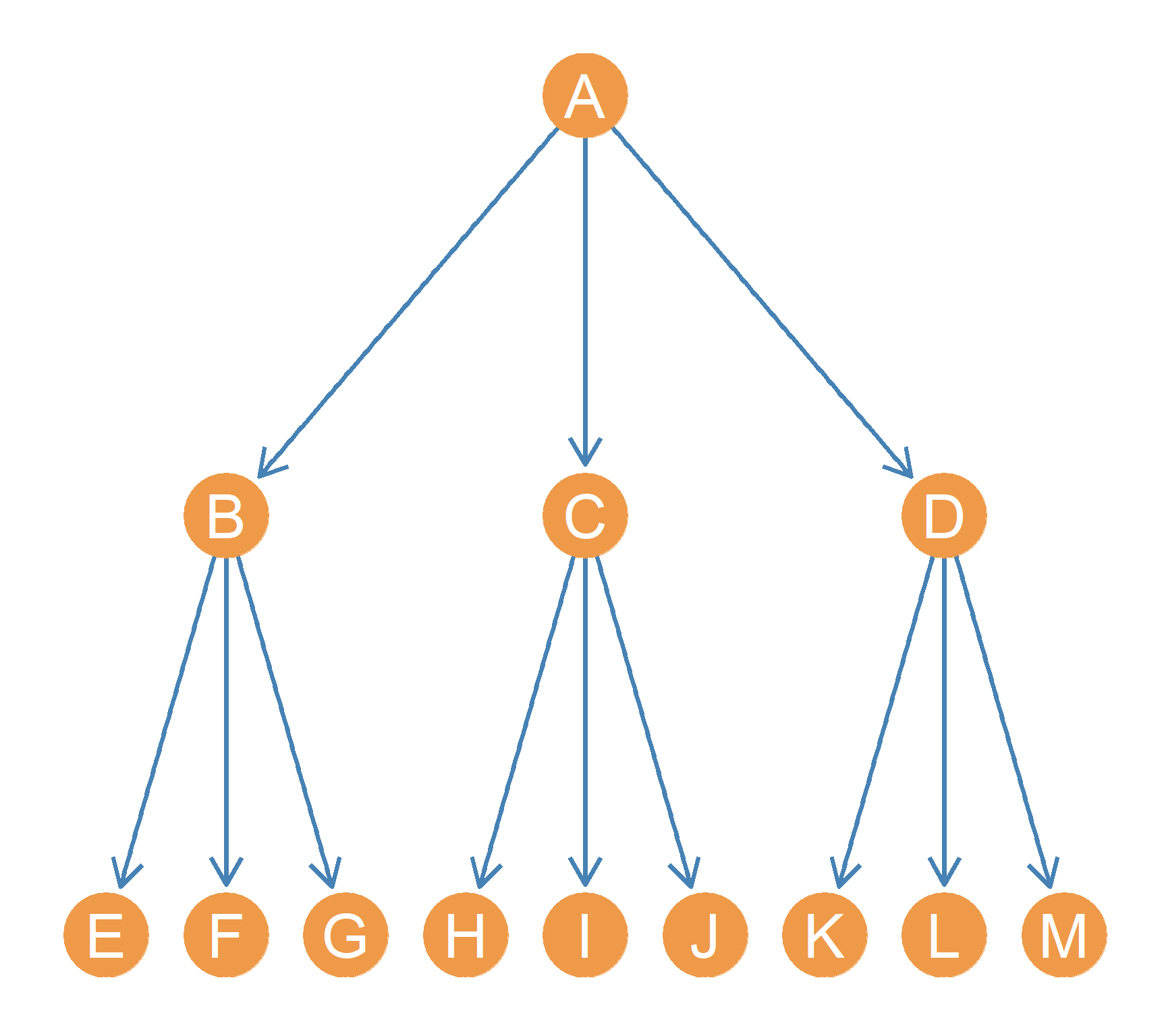Figure 3.1: A tree graph.

One feature of a network composed of only anti-symmetric relations is that its corresponding graph can always be drawn from top to bottom, starting (at the top) with the node that only sends but does receive any ties and ending (at the bottom) with nodes that only receive, but do not send, ties. This is called a tree graph and an example is shown in Figure 3.1. Your family tree is an example of a tree graph of anti-symmetric kin ties. For instance, A could be your grandmother, and B, C, and D could be her three daughters. If B was you mom, then you could be E (along with your siblings F and G) and your cousins H, I, J, K, L, M.

Teacher-student, coach-athlete, buyer-seller are all examples of anti-symmetric relationships that can be depicted as tree graphs. In a future lesson, we will see that it is possible to characterize the level of anti-symmetry we observe in a directed graph, to see how closely they approximate the pure tree structure.

In addition to kinship, authority relations are a common antisymmetric tie between people. Thus, Figure 3.1 could be a network in which the anti-symmetric links are directed “gives orders to” (in an army or an office) relations, where the source node directs commands toward the destination node. So A is the top boss and commands B, C, and D. Node B, in their turn, gives orders to E, who is at the lowest level of the hierarchy, not commanding anybody in turn.

4 Tie Strength and Weighted Graphs

In the preceding sections, our understanding of relationships have centered around their existence or absence. In certain situations, it may be socially meaningful to consider relationships in terms of their intensity or frequency- or what is often referred to as the “strength” of the tie (Marsden and Campbell 1984Marsden, Peter V, and Karen E Campbell. 1984. “Measuring Tie Strength.” Social Forces 63 (2): 482–501.).

For example, people might have many friends, and friendship ties can be turned into graphs as done in the above examples. However, people often have different types of friends, and some friends are more important than others. This is the idea behind the concept of having a best friend. Your best friend might be more important to you than all your other friends, and it might be sociologically meaningful to the topic you are studying to capture this difference in your network. While it might be a little meaningless to just mark your best friend different from the rest, we can think of social situations where a series of gradations might make sense.

For example, let’s say that we want to understand who is the leader in a group of friends. By definition, we’ve already bound the case as an existing group of friends. If everyone had a tie to the others, because they’re all friends, then we would not be able to detect any variation between these different friends. However, if we looked at the frequency of text messages sent from one of these friends to another one, then we would likely begin to detect variation.

Figure 4.1: An undirected weighted graph.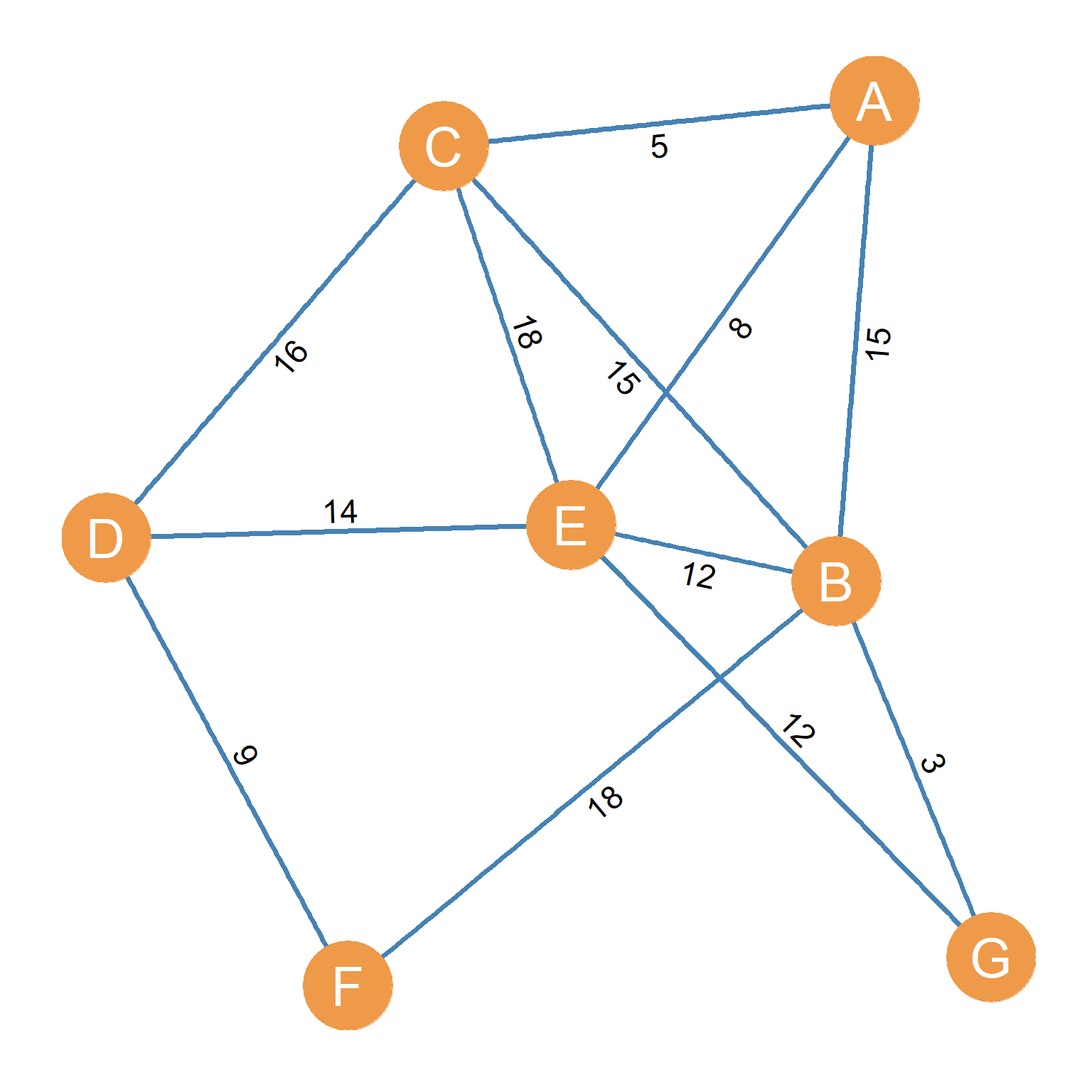The variation in the strength of these ties in social networks is captured by using weighted graphs to represent such networks. Figure 4.1 shows an example of a undirected weighted graph. In weighted graphs, the relative intensity of the relationships between actors in the network is quantified, thus facilitating the comparison of particular actors and relationships within the network. This is done by associating each edge in the graph with a number, call the weight of that edge. So instead of being just a set of vertices and edges, a weighted graph ($$G_w$$) is a set of three sets. A set of vertices ($$V$$), a set of edges ($$E$$), and a set of weights ($$w$$) associated with each edge:

$\begin{equation} G_w = (E, V, w) \end{equation}$

Thinking of Figure 4.1 as a type of undirected tie, the numbers can be thought of as the intensity of the link between two people. Perhaps these are the number of times two people have met for coffee or a drink during last year. Inspecting the Figure, we can see that actors C and E hang out together quite frequently (perhaps they are close, or are working on a project together). Actors B and G, on the other hand, hang out together less often.

Actors also seem to have preferences as to which people they hang out with most frequently with among those they are connected to. For instance, actor C has four contacts in the network. However, they have met only a few times with A but meet quite a lot times with their other contacts. This means that C has a weak tie with A and a strong tie to the rest of their friends.

Figure 4.2: A directed weighted graph.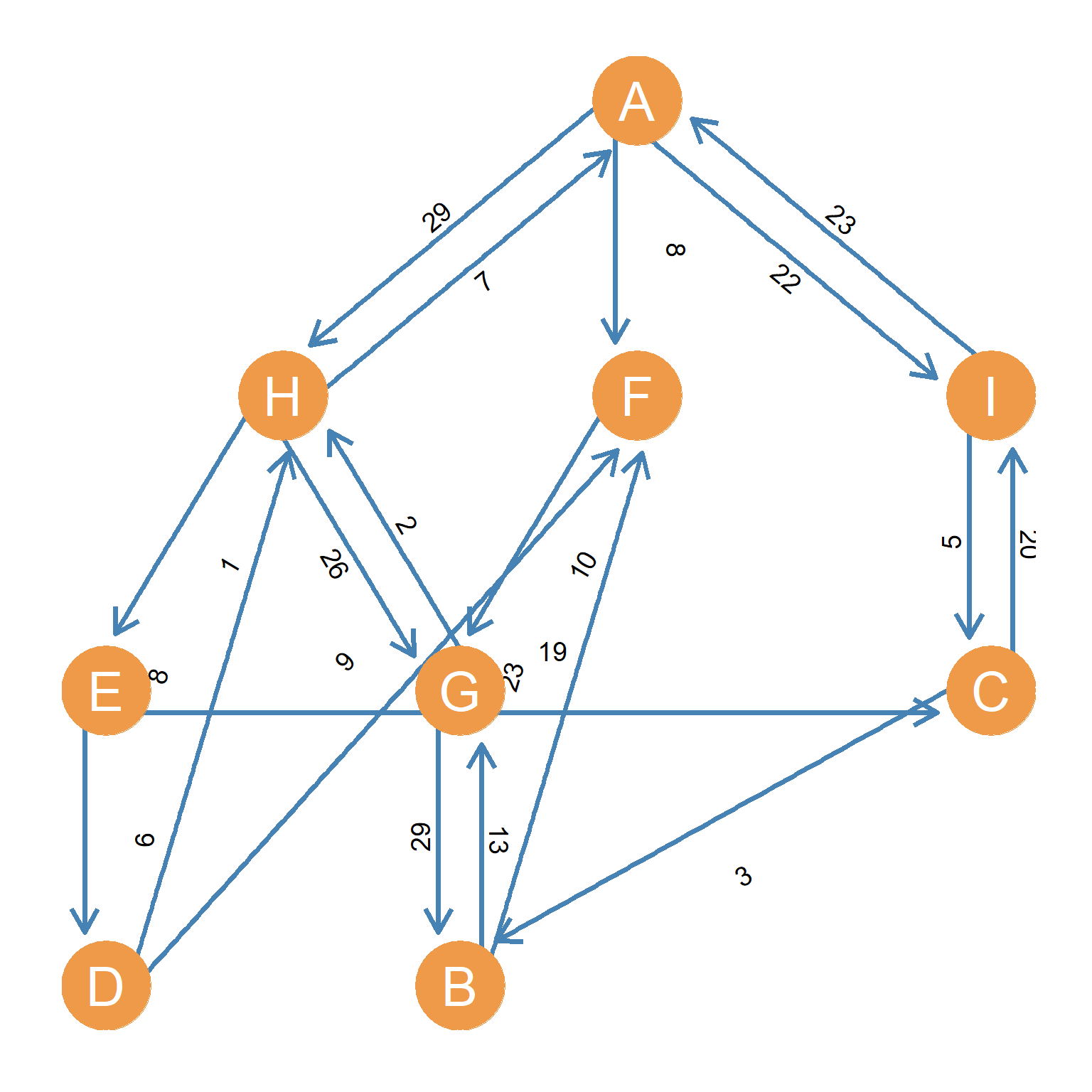Weighted graphs can also be directed, like the one shown in Figure 4.2. The number along each asymmetric tie could be the number of times one actor calls or texts the other, or the number of times they retweet the other other person, or the number of times they like a post from the other person on Instagram. Note that all of these things can lead to imbalance, such that in weighted graphs, relationships can be non-reciprocal even if the two actors are connected bi-directionally (as we will see in a future lesson). For instance, C directs an edge of weight $$w= 20$$ towards I (perhaps a number of texts), but I only sends a directed edge of weight $$w = 5$$ towards C.

From varying weights among edges in a social network, representing some varying frequency or intensity of the relationship in the real world, we can better understand important sociological phenomena.

5 Sentiment Relations and Signed Graphs

5.1 Sentiment Networks

So far we have talked about social relations as having different properties above and beyond being either “on” or “off.” Social relations can be weak or strong or they can be multiplex or uniplex. Another property that social relations can have is valence. That is, you can be connected to other people via either positive or negative links.

For instance, you can love or hate someone. You can like or dislike a person. Somebody can consider you their enemy or their friend. A terrible person can bully you, or you a kind person can help you. What all of these contrasts in connectivity have is that they distinguish relations by their valence, and that valence takes on one of two possible values.3 Relations that can take one of two opposed values are called bipolar. Social Networks that are composed of valenced relationships are called sentiment networks.

Can you think of other examples of sentiment networks you have experience with?

5.2 Signed Graphs

As you might have already suspected, there is a special type of graph that is useful for representing sentiment networks. This is called a signed graph. An example of a signed graph is shown in Figure 5.1. This signed graph is complete because all the possible relations between nodes exist. Note also that the signed graph is directed because each node is both a source and a destination node for directed asymmetric ties.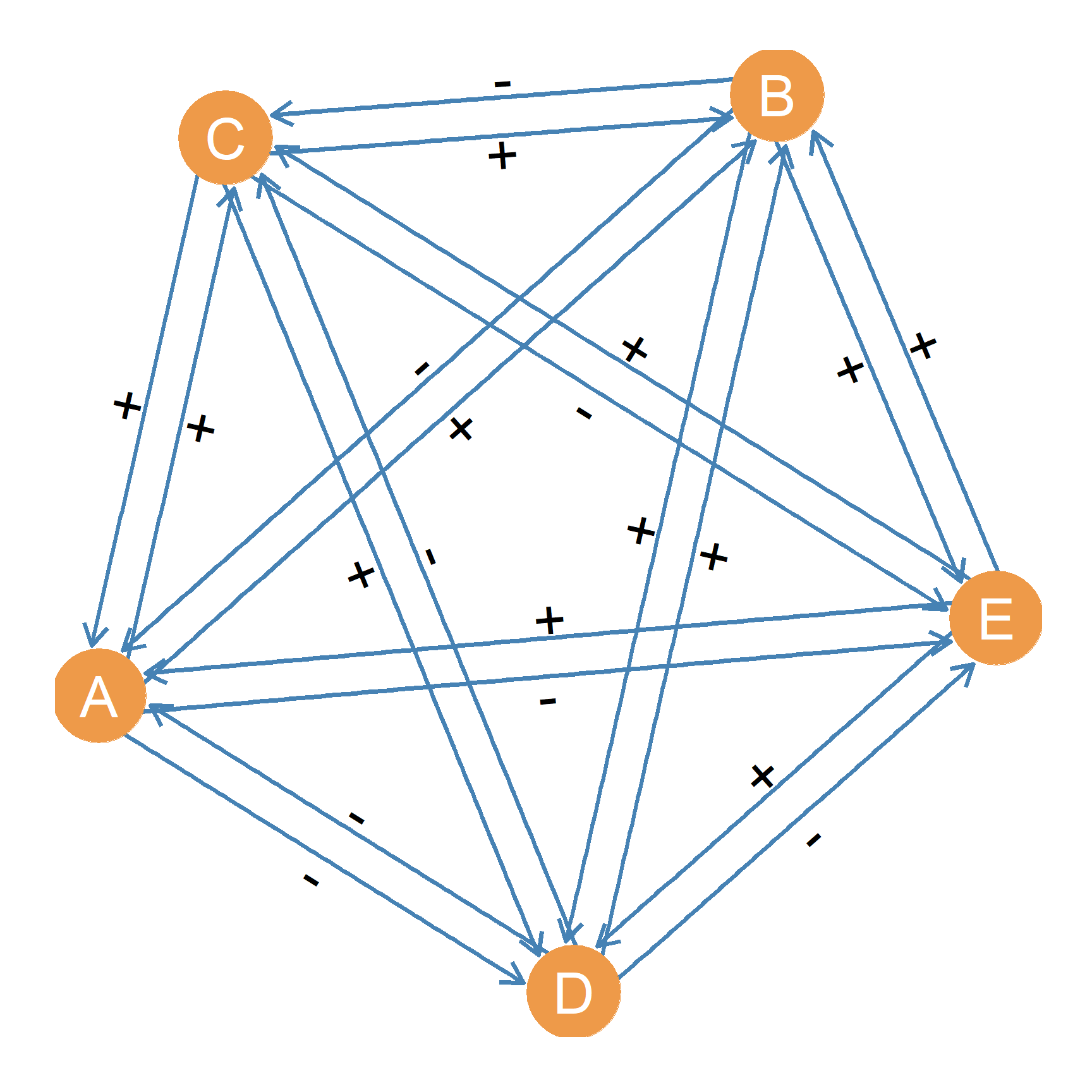Figure 5.1: A directed signed graph.

Mathematically, one way to think about a signed graph is as a special kind of multigraph ($$G_S$$) featuring two disjointsets of edges4 Two sets $$A$$ and $$B$$ are disjoint when they don’t share any members. That means their intersection is the empty set: \$A B = : positive links ($$E^+$$) and negative links ($$E^-$$). Thus, a signed graph is a set of three sets:

$\begin{equation} G_S = (E^+, E^-, V) \end{equation}$

Signed graphs have a number of unique properties. Some of them are the basis for entire network theories, such as balance theory, status theory, and karma theory that we will discuss later. For instance, reciprocity, just as in weighted graphs, takes on a different meaning in complete signed graphs. In the usual graph theory sense, all the relations in 5.1 are “reciprocal” because the graph is complete and thus all the dyads are mutual.

However, in complete signed graphs, reciprocity is better defined as mutual dyads that have the same sentiment going from one node to the other. In a signed graph mutual dyad, the relationship is reciprocal if both people think that they are friends or both people hate one another. A mutual dyad in a signed graph is non-reciprocal if one person likes the other person, but that person hates the first person. In the graph theoretic sense, reciprocal dyads in a signed graph are those that are connected by two asymmetric edges of the type: either positive or negative. A dyad is non-reciprocal if the two nodes are connected by asymmetric edges of different types.

Thus, in 5.1 Nodes A and C have a mutually positive relationship; A likes C and C reciprocates by liking A back. In the same way, A and D have a mutually negative relationship; A hates D and D reciprocates by hating A back. While the notion of “reciprocity” in negative interactions like hating, or bullying seems counter-intuitive (because we tend to think of reciprocity as an inherently positive thing), we will see later, when discussing theories of negative interactions, that negative reciprocity makes sense as a driver of human behavior, and may explain important phenomena like the escalation of violence among urban gangs (Papachristos, Hureau, and Braga 2013Papachristos, Andrew V, David M Hureau, and Anthony A Braga. 2013. “The Corner and the Crew: The Influence of Geography and Social Networks on Gang Violence.” American Sociological Review 78 (3): 417–47.).

Finally, note that nodes B and C have a non-reciprocal sentiment relation; B likes C but C does not reciprocate the sentiment. Instead, C dislikes B. This brings us to another property of signed graphs, which is that this type of “imbalance” makes us think that there is something wrong with this dyadic state, and that something will have to give. Either C starts to hate B (because their feelings are hurt), or C ultimately convinces B to like them back.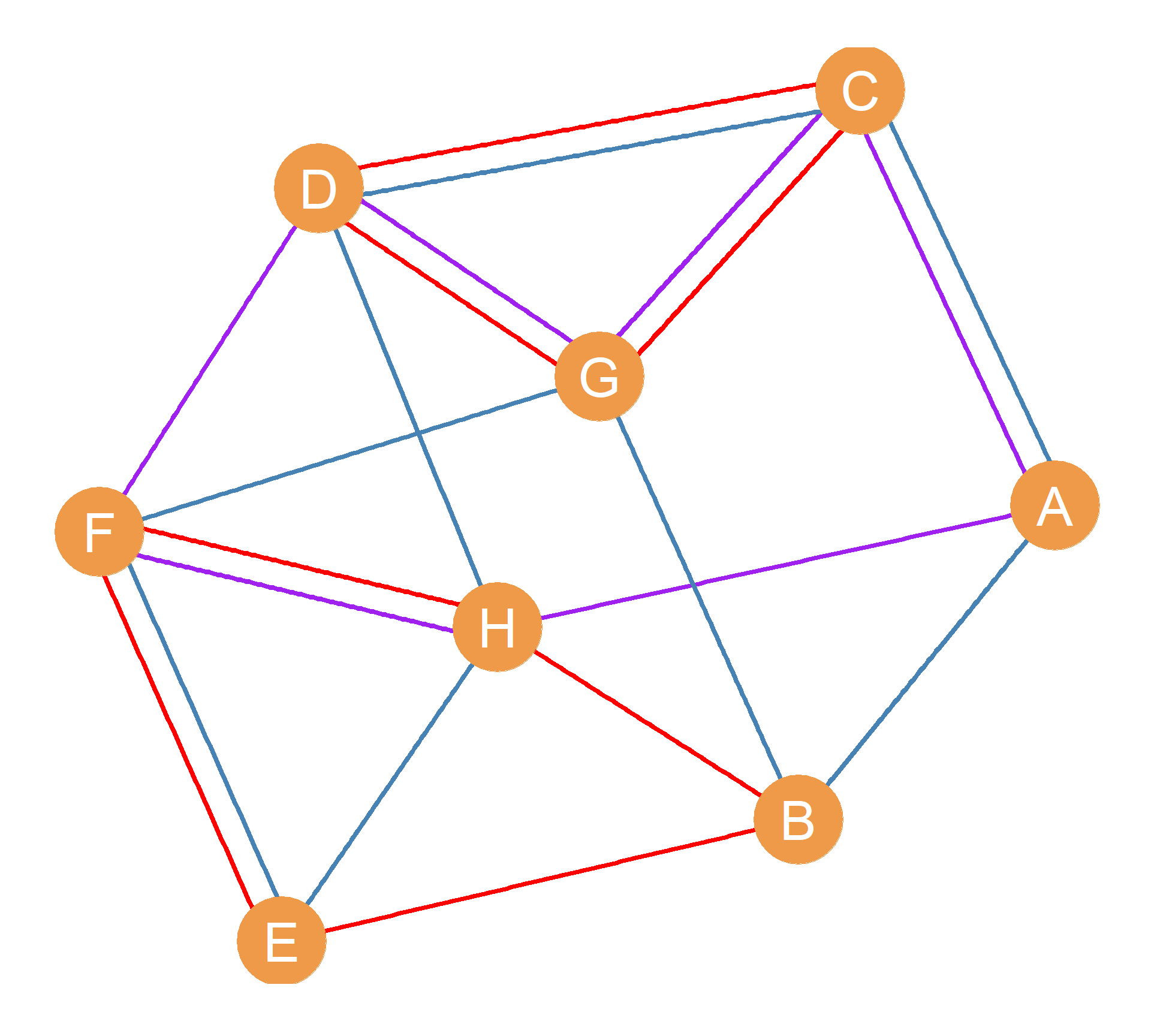Figure 5.2: An undirected multigraph.
Multiplexity, as a common feature of social life, can be represented using a special type of graph called a multigraph. A multiple graph ($$G_M$$) is just like a regular graph, except that instead of having a single edge set $$E$$, it has multiple edge sets $$(E_1, E_2,\dots V_K$$), where $$K$$ is the total number of different types of relations in the network:
$\begin{equation} G_M = (E_1, E_2,\dots E_K, V) \end{equation}$
A network diagram of a multigraph is shown in Figure 5.2. This graph has eight nodes joined by three different types of ties ($$K = 3$$). The ties in a multigraph are labeled so that we can tell the different kinds apart. In the figure, the type-of-tie labels are represented by different edge colors. For instance, if the three relations we are studying are friendship (blue), co-working (red), and being a member of the same soccer club (green), then we can see nodes D and C are a multiplex dyad because are connected in two distinct ways (they are co-workers and members of the soccer club). Nodes C A are also a multiplex dyad because they are friends who also happen to work together. Nodes B and H, by way of contrast, are a regular old uniplex dyad being connected by a single type of tie (they are both in the soccer club, but do not work together nor do they think of one another as friends). The same goes for the dyad formed by nodes D and F who are just friends who neither work together nor belong to the same club. Finally, note that in Figure 5.2 nodes A and D are part of a regular old null dyad (they are not connected by any type of relation).